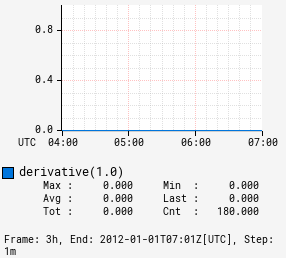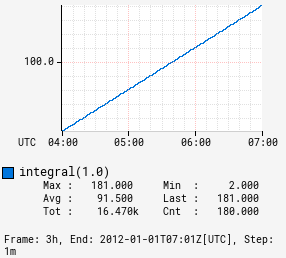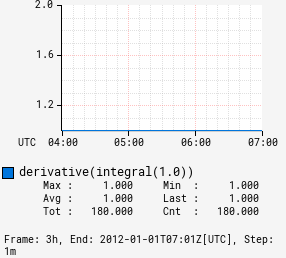# derivative

Input Stack:
 TimeSeriesExpr
Output Stack:
 TimeSeriesExpr

Opposite of :integral. Computes the rate of change per step of the input time series.

 Derivative Integral Integral Then Derivative```1,:derivative ``` ```1,:integral ``` ```1,:integral, :derivative ```